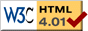# Mutual Attraction

previous next
• Sim
• Graph
• Time Graph
• Multi Graph

Physics-based simulation of objects under an inverse square gravity law using the 2D Rigid Body Physics Engine.

Change parameters such as gravity, damping, elasticity of collisions, and number of objects. Drag objects by applying a spring force with your mouse.

The objects are all mutually attracting with the force given by

f = G m1 m2 / r2

where G is the gravitational constant, m1 and m2 are the mass of the two objects and r is the distance between them.

Also available are: open source code, documentation and a simple-compiled version which is more customizable.

This web page was first published January 2011.

previous next